## Introduction

Symmetry plays a critical role in the scientific and mathematical descriptions of the universe. Symmetries can be continuous, as in rotations of a circular cylinder about its axis; or discrete, as in end-for-end exchanges of the cylinder about its midpoint. Discrete polytope symmetries appear in diverse and widespread systems, including crystals, molecular bonds, and the familiar morphologies of honeycombs, snowflakes, and flower petals. They can also be hidden in the internal states of otherwise continuous and isotropic systems, where they can have profound and unusual consequences; for example, the discrete symmetries of charge conjugation, parity, and time-reversal play important roles in particle and condensed matter physics and serve as a touchstone for grand unified theories. Complex discrete symmetries also appear in spatially uniform condensed matter systems and, intriguingly, in spinor superfluids.

In quantum mechanics, the internal symmetries of a spin-F system can be conveniently described in a geometrical representation due to Majorana, wherein a state corresponds to a constellation of 2F points on the unit sphere1,2. Each point is related to the state of an independent spin-1/2 system3, and the polytopes with vertices established by the representative points display the discrete symmetries of the order parameter that describes the system4,5,6. The stationary, dynamically stable states that share a given constellation are known as magnetic phases, whose richness of internal symmetries is illustrated by the examples in Fig. 1.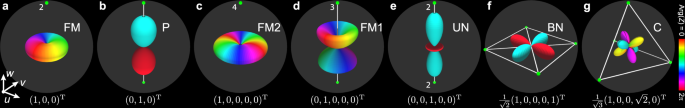Fig. 1: Majorana and spherical-harmonics representations of the prototype spinors for spin-1 and spin-2 magnetic phases.

In contrast to the ubiquity of their crystalline counterparts, condensed matter systems exhibiting discrete polytope symmetries within their internal degrees of freedom are relatively unusual, with examples appearing in exotic contexts such as d-wave superconductors7, 3P2 neutron star superfluids8, and biaxial nematic liquid crystals9. Spinor Bose–Einstein condensates (BECs)10 with spin F ≥ 2 provide an exciting pristine system with unprecedented experimental control. They are described by an order parameter whose complex broken internal symmetries lead to magnetic phases with polygonal (e.g., Fig. 1f), tetrahedral (e.g., Fig. 1g), octahedral (F ≥ 3), and icosahedral (F ≥ 6) Majorana symmetries. Previous experimental studies have examined phenomena associated with spinor BECs in simple magnetic phases that lack such symmetries, including topological defects11,12,13,14 and textures15,16,17,18,19, spontaneous pattern formation20,21, dipolar interactions22, magnons23, and condensate fragmentation24. However, apart from early experiments on spin population dynamics25, magnetic phases with discrete polytope symmetries remain unexplored.

Here, we create and verify the discrete polytope symmetries of biaxial nematic (BN) and cyclic (C) phases of spin-2 BECs in the rotational properties of their continuous wave functions and in the singular line defects. The BN and C magnetic phases exhibit discrete internal Majorana symmetries of a square (Fig. 1f) and a tetrahedron (Fig. 1g), respectively, which are revealed experimentally. Such phases support exotic singular vortices that we prepare using combinations of magnetic field rotations and carefully engineered microwave transitions. The singular defect cores are filled by a variety of different superfluid phases, resulting in rich combinations of core structures that form interfaces between different discrete and continuous symmetries. Our experimental procedure thus establishes a fascinating setting for the exploration and manipulation of the unusual properties of the line defects26,27,28,29,30 that directly emerge from the discrete polytope symmetries. For instance, the line defects may not commute when the vortex positions are interchanged, leaving rung vortices behind in collisions30,31 with possible ramifications for interferometry and quantum information32.

## Results

We create line defects by applying a carefully tailored time- and spatially varying magnetic field to a spin-1 87Rb superfluid in its ferromagnetic (FM) phase, which maximizes the spin magnitude, with the order parameter defined by spatial rotations (Fig. 1a). The changing magnetic field imprints a nonsingular vortex texture with a tight bending of the magnetization in the vicinity of its core, triggering an instability that induces the system to decay into a pair of singly-quantized, singular vortices in the FM phase13,33. During the decay process the vortex cores fill with superfluid in the polar (P) magnetic phase, with nematic symmetry (Fig. 1b) and vanishing condensate spin magnitude. The magnetic spin-2 superfluid phases are subsequently introduced with a sequence of microwave pulses that promote the spin-1 atoms into the spin-2 hyperfine manifold. The result is a pair of line defects in any of the prototypical spin-2 magnetic phases shown in Fig. 1c–g, but we focus here on converting the FM phase to either the BN or C phase that display discrete polytope symmetries. In the same pulse sequence, the filled core in the P phase is similarly converted to a distinct F = 2 magnetic phase of our choice, such as ferromagnetic-2 (FM2), with maximal spin magnitude and symmetry related to the spatial rotations, or uniaxial nematic (UN), with vanishing condensate spin and the symmetry defined by an unoriented axis and a 2π change of the global BEC phase. (Further details of the magnetic phases are provided in the Methods and in Supplementary Fig. 1.)

Our experimental creation technique accesses an entire family of line defects in which the polytope symmetries affect the properties of the vortex cores. An example of a microwave pulse sequence that yields a pair of BN vortices with filled UN cores is shown in Fig. 2a. In the chosen basis the BN condensate is represented by a square in the uv- (horizontal) plane of the Majorana sphere (Fig. 1f), whereas the UN core is similarly represented by a rod oriented along the w- (vertical) axis (Fig. 1e). More generally, changing the relative phase between the nonzero spinor components of either the BN or C phases (Fig. 1f, g) amounts to rotating the corresponding polytope about the w-axis, and is achieved experimentally by selecting the appropriate phases of the microwave pulses.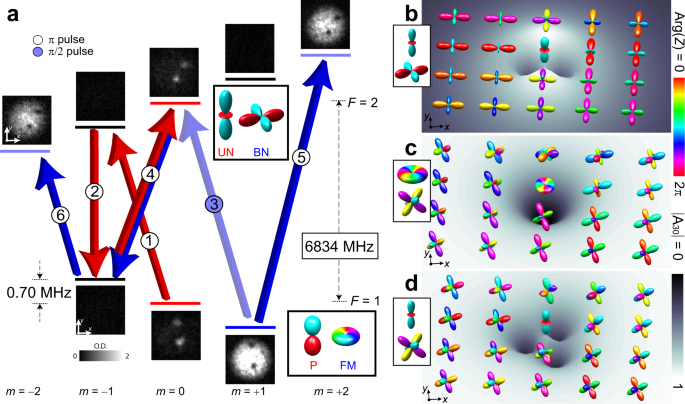Fig. 2: Creation of singular line defects in magnetic phases with discrete polytope symmetries.

The formation of the vortex core can be understood in this example of the singly-quantized BN line defect as the continuous transformation of the BN bulk phase towards the UN phase inside the vortex core (Fig. 2b). We can parameterize the stationary spinor solution by

$$\zeta=\left(\begin{array}{l}{e}^{i\phi }f(\rho )/\sqrt{2}\\ 0\\ \sqrt{1-{[f(\rho )]}^{2}}\\ 0\\ {e}^{i\phi }f(\rho )/\sqrt{2}\end{array}\right)\,,$$
(1)

where f(ρ) parameterizes the radial vortex-core profile in terms of the radial coordinate $$\rho={({x}^{2}+{y}^{2})}^{1/2}$$, such that f(ρ) → 1 outside the core, and f(ρ) = 0 on the line singularity itself (ρ = 0). This solution smoothly interpolates between the BN and UN phases. Hence, the total superfluid density remains non-zero as the vortex line is filled with the UN phase, even though the BN phase becomes singular and must vanish. This is unlike a defect singularity in a scalar superfluid that has a vanishing superfluid density. The solution given by Eq. (1) thus constitutes a stable, coherent topological interface at the vortex core33,34. Vortex cores with complex internal structures in the absence of discrete polytope symmetries have been extensively studied in superfluid liquid 3He35 and also in atomic spinor condensates36. Additionally, topological interfaces more generally can support non-trivial topological objects whose classification changes as they penetrate the interface. These have been extensively studied, e.g., in high-energy physics and cosmology37,38, in superfluid liquid 3He39, and in BECs34.

Further instructive examples are illustrated in Fig. 2c, d, in which the core of a C line defect is filled with either the FM2 or the UN phase (see Supplementary Figs. 24 for additional experimental realizations). The Majorana representation of the C phase in these cases yields a vertex-up tetrahedron with a face parallel to the uv-plane. While the FM2 core is rotationally symmetric, the core of the resulting singly-quantized C vortex is remarkably anisotropic when the UN phase occupies the line singularity. Numerical simulations show that the vortices in both cases are unstable against splitting into a pair of vortices carrying fractional $$\frac{1}{3}$$ and $$\frac{2}{3}$$ circulation quanta, respectively. In the process, the $$\frac{2}{3}$$ vortex develops a FM2 core, while the core of the $$\frac{1}{3}$$ vortex exhibits the ferromagnetic-1 (FM1) phase (cf., Fig. 1c, d). However, the decay is sensitive to the orientation of the order parameter of the imprinted vortex. In simulations, we find that a π/4 spin rotation about the v axis instead causes a decay of the condensate into the BN phase, forming vortices with FM2 cores.

Images of the condensate spinor components reveal the rich structure of line defects in the magnetic phases with polytope symmetries but do not directly show the discrete internal symmetries of the phases in real space. To verify these symmetries we apply radio-frequency (rf) spin-tip pulses that rotate the entire Majorana constellation about an axis in the uv-plane1, thereby preserving the magnetic phase but changing its spinor representation. The rotation axis and angle of rotation are established by the phase and area of the rf pulse, respectively, in the same fashion as they act on states on the Bloch sphere2. Critically, the spinor component densities remain invariant under any 2π/j adjustments of the rf phase along a j-fold symmetry axis of the polytope.

Extending our previous example, we demonstrate the three-fold discrete symmetry of the cyclic magnetic phase by rotating the vertex-up tetrahedral state of the C phase (Fig. 1g) through the tetrahedral angle $$\arccos (1/\sqrt{3})$$ about the axis in the uv-plane established by the phase of the spin-tip pulse. As the rf phase is varied through $$\frac{2\pi }{3}$$ radians (Fig. 3b–e), the final vortex state undergoes one corresponding revolution that returns to the edge-up state $$\frac{1}{2}{(1,\;0,\;i\sqrt{2},\;0,\;1)}^{T}$$, as shown in Fig. 3b, d. The imaginary amplitude of the m = 0 component, inferred from the measured spinor component densities after the rotation, is critical to distinguishing the cyclic magnetic phase from a mixed UN-BN phase that has the same spinor component densities but does not share the tetrahedral Majorana symmetry. Similar measurements reveal the four-fold Majorana symmetry of the BN phase under π/2 rotations (Fig. 4) and the full three-fold symmetry of the C phase under 2π/3 rotations (Supplementary Fig. 5).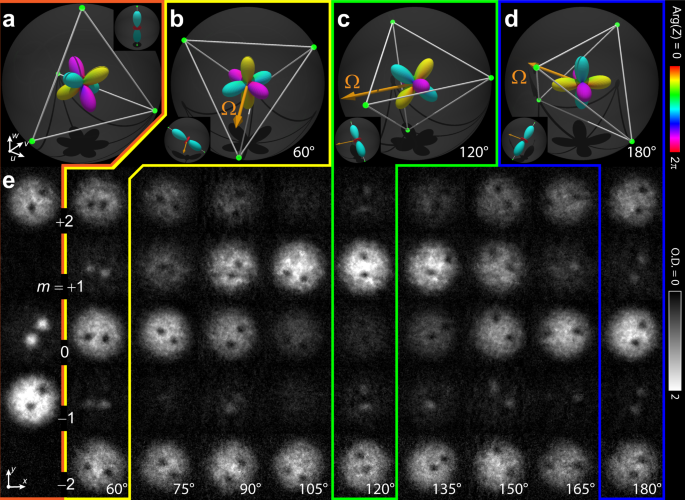Fig. 3: Demonstration of tetrahedral discrete symmetry in the continuous wave function of the spin-2 superfluid in the cyclic phase with a singular line defect.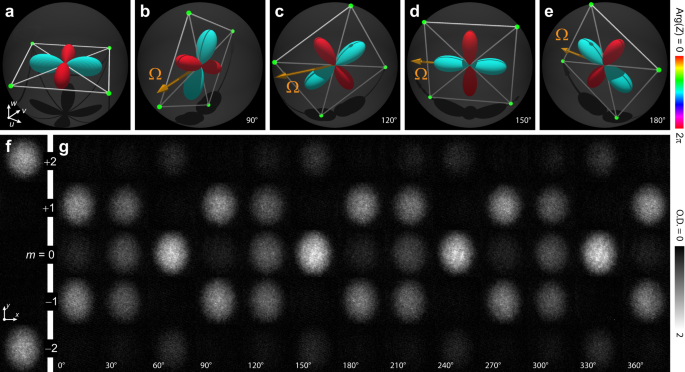Fig. 4: Demonstration of polytope discrete symmetry in the continuous wave function of the spin-2 superfluid in the biaxial nematic phase.

## Discussion

Our creation of line defects in magnetic phases with demonstrated polytope internal symmetries suggests a number of future experiments. As a proof of principle, we experimentally explored the time evolution of a UN core, C vortex condensate for the first 30 ms after a tetrahedral rf rotation into the edge-up tetrahedral state (Figs. 3b, d and 5). Over this interval, the total number of atoms decreases substantially, whereas the fraction of atoms in each spinor component changes only slightly: the m = ± 1 spinor components become more diffuse in the region of the vortex core, and the fraction of atoms in the m = 0 component diminishes slightly with respect to the m = ± 2 spinor components. These results suggest that the C superfluid trends slowly towards the BN phase, consistent with previous experimental studies25. The relatively short superfluid lifetime is a constraint that can be addressed with the development of optical trapping geometries that reduce the atomic density. Overcoming this technical hurdle will therefore establish a path to exploring the vortex dynamics more fully, including the expected evolution towards fractionally-quantized vortices we highlighted above.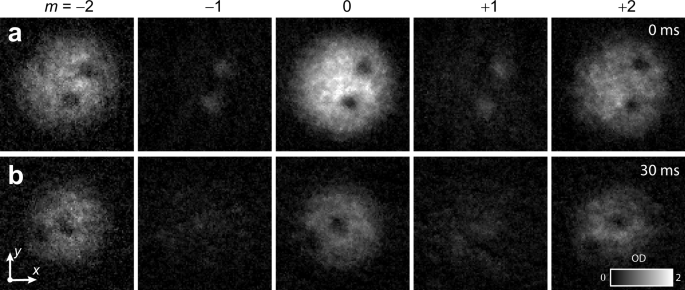Fig. 5: Initial time evolution of the singular line defects in the cyclic phase, with the defect core in the uniaxial nematic phase.

Our engineering of magnetic phases with discrete polytope symmetries and the associated line defects also opens new avenues for the further exploration of exciting phenomena that have previously only been associated with exotic models in field theories of high-energy physics and cosmology. For instance, defects across the interfaces of polytope symmetric magnetic phases can combine non-trivially, forming singularities that penetrate continuous, coherent topological interfaces and non-Abelian line defects appear as cosmic strings in theories of the early Universe40. Non-Abelian defects in the magnetic phases of discrete polytope symmetries may furthermore form a non-commutative algebra26,27,28,29,30 that could potentially be harnessed for quantum information processing32. Experiments for manipulating such defects are challenging but specific proposals for preparing pairs of non-commuting vortices already exist30. Experimental manipulation may also be assisted by working in, e.g., strongly oblate trapping geometries where the defects become more easily controllable point vortices.

## Methods

### Spin-2 Hamiltonian

We represent the macroscopic spin-2 BEC wave function as $${{\Psi }}({{{{{{{\bf{r}}}}}}}},\;t)=\sqrt{n({{{{{{{\bf{r}}}}}}}},\;t)}{e}^{i\tau }\zeta$$, such that the atom density n = Ψ2, the global phase τ(r, t), and the spinor ζ(r, t) are governed by a mean-field Hamiltonian density10

$${{{{{{{\mathcal{H}}}}}}}}= \frac{{\hslash }^{2}}{2{M}_{{{{{{{{\rm{a}}}}}}}}}}|\nabla {{\Psi }}{|}^{2}+{U}_{{{{{{{{\rm{trap}}}}}}}}}n+\frac{{c}_{0}}{2}{n}^{2}+\frac{{c}_{1}}{2}{n}^{2}|\langle \hat{{{{{{{{\bf{F}}}}}}}}}\rangle {|}^{2}\\ +\frac{{c}_{2}}{2}{n}^{2}|{A}_{20}{|}^{2}+pn\langle {\hat{F}}_{z}\rangle+qn\langle {\hat{F}}_{z}^{2}\rangle$$
(2)

in the harmonic trapping potential $${U}_{{{{{{{{\rm{trap}}}}}}}}}=({M}_{{{{{{{{\rm{a}}}}}}}}}{\omega }_{r}^{2}/2)({x}^{2}+{y}^{2}+2{z}^{2})$$, with radial trap frequency ωr. Here, Ma denotes the atomic mass, $${A}_{20}=\frac{1}{\sqrt{5}}(2{\zeta }_{+2}{\zeta }_{-2}-2{\zeta }_{+1}{\zeta }_{-1}+{\zeta }_{0}^{2})$$ is the amplitude of spin-singlet pair formation, and p and q determine, respectively, the linear and quadratic Zeeman shifts. The condensate-spin expectation value $$\langle \hat{{{{{{{{\bf{F}}}}}}}}}\rangle={\sum }_{\alpha \beta }{\zeta }_{\alpha }^{{{{\dagger}}} }{\hat{{{{{{{{\bf{F}}}}}}}}}}_{\alpha \beta }{\zeta }_{\beta }$$ is obtained from the vector $$\hat{{{{{{{{\bf{F}}}}}}}}}$$ of spin-2 matrices. The nonlinearities c0 = 4π2(3a4 + 4a2)/7Ma, c1 = 4π2(a4 − a2)/7Ma, and c2 = 4π2(3a4 − 10a2 + 7a0)/7Ma are derived from the s-wave scattering lengths as for the total spin s of the interacting atom pairs, a0 = 87.4(10)aB, a2 = 92.4(10)aB, and a4 = 100.5(10)aB in units of the Bohr magneton aB41.

For a weak quadratic Zeeman shift, the spin-2 BEC exhibits different magnetic phases that are characterized by the values of $$|\langle \hat{{{{{{{{\bf{F}}}}}}}}}\rangle|$$ and A20. For a ferromagnetic phase, A20 = 0 and $$|\langle \hat{{{{{{{{\bf{F}}}}}}}}}\rangle \vert=2$$ (for FM2) or 1 (for FM1). The representative spinors are obtained by rotations of (1, 0, 0, 0, 0)T and (0, 1, 0, 0, 0)T, respectively. For the C phase, $$|\langle \hat{{{{{{{{\bf{F}}}}}}}}}\rangle \vert=\vert {A}_{20} \vert=0$$ and the spinor representations are obtained by rotations of $$\frac{1}{\sqrt{3}}{(1,\,0,\,0,\,\sqrt{2},\,0)}^{T}$$. For spin-2 BECs, the polar magnetic phase, which also has a vanishing spin magnitude $$|\langle \hat{{{{{{{{\bf{F}}}}}}}}}\rangle \vert=0$$ but $$|{A}_{20} \vert=\frac{1}{\sqrt{5}}$$, is found to separate into two distinct phases: UN and BN, represented by the spinors (0, 0, 1, 0, 0)T and $$\frac{1}{\sqrt{2}}{(1,\,0,\,0,\,0,\,1)}^{T}$$, respectively. By defining the amplitude of spin-singlet trio formation10 $${A}_{30}=\frac{3\sqrt{6}}{2}({\zeta }_{+1}^{2}{\zeta }_{-2}+{\zeta }_{-1}^{2}{\zeta }_{+2})+{\zeta }_{0}(-6{\zeta }_{+2}{\zeta }_{-2}-3{\zeta }_{+1}{\zeta }_{-1}+{\zeta }_{0}^{2})$$ we can distinguish between the UN and BN phases that take the values A30 = 1 and 0, respectively. For the C phase $$|{A}_{30} \vert=\sqrt{2}$$.

We solve the five coupled Gross-Pitaevskii equations obtained from Eq. (2) numerically using a split-step method42 and experimental parameter values. Angular momentum is conserved in s-wave scattering, implying that on time scales where this dominates, longitudinal magnetization, Mz = (1/N)∫d3rn(r)Fz(r), where N is the total number of atoms, is conserved. We employ an algorithm that explicitly conserves Mz.

### Graphical illustration of magnetic phase symmetries

We represent the order-parameter symmetry of the magnetic phases in terms of the complementary spherical-harmonics and Majorana representations. The stars of the Majorana constellation1 are calculated by numerically finding the 2F + 1 roots z = zj of the complex polynomial,

$$\mathop{\sum }\limits_{\alpha=0}^{2F}\sqrt{\left(\begin{array}{l}2F\\ \alpha \end{array}\right)}\,{\zeta }_{F-\alpha }^{*}{z}^{\alpha }=0,$$
(3)

where each root then represents a stereographic projection $${z}_{j}=\tan \left(\frac{\theta }{2}\right){e}^{i\phi }$$ of the spherical coordinates (θ, ϕ) that define the Majorana points. Rotations of the state are equivalent to rotations of the constellation on the Majorana sphere that preserve their relative orientation. In the spherical harmonics representation we show Z(θ, ϕ)2, where $$Z(\theta,\; \phi )=\mathop{\sum }\nolimits_{m=-F}^{+F}{Y}_{F,m}(\theta,\;\phi ){\zeta }_{m}$$ expands the state in terms of the spherical harmonics YF,m(θ, ϕ) (F = 1, 2). The symmetry is completed by the color scale that is obtained from $${{{{{{{\rm{Arg}}}}}}}}(Z)$$. The spherical-harmonics representation can be obtained by representing the spin-F condensate wavefunction in terms of a complex polynomial of degree F28.

### Magnetic phases and singular vortex lines

The spin-2 FM2/1 and UN phases are closely related to the spin-1 ferromagnetic (FM) and polar (P) phases, where the order parameter of the FM phase corresponds to the group of spatial rotations, SO(3). The singular line defects in such a system, as in the FM1 phase of spin-2, can only belong to two topologically distinct equivalence classes, representing the winding numbers zero and one13. The FM2 phase in the spin-2 system doubles the number of these classes to four. The UN phase is determined by the nematic axis $$\hat{{{{{{{{\bf{d}}}}}}}}}$$ and the global phase of the macroscopic condensate wave function τ. The order parameter symmetry is $${S}^{1}\times {S}^{2}/{{\mathbb{Z}}}_{2}$$, where the two-element group factorization is due to the identity of the states $$\zeta (\hat{{{{{{{{\bf{d}}}}}}}}},\;\tau )=\zeta (-\hat{{{{{{{{\bf{d}}}}}}}}},\;\tau )$$. This nematic symmetry allows for the existence of spin half-quantum vortices (HQVs).

The BN and C phases exhibit polytope symmetries, as shown in Fig. 1f–g, that result in a much richer structure of singular line defects. The topologically distinct families of line defects in such magnetic phases derive from the conjugacy classes of the fundamental homotopy group π1 of the corresponding order-parameter space symmetries. Specifically, these are determined directly from the group of transformations that leave the order parameter unchanged43—here by applying a SO(3) spin rotation and a S1 gauge transformation by the global condensate phase τ. For the case of the BN phase, the fourfold symmetry of the Majorana constellation is illustrated in Fig. 1f. Transformations that exactly interchange the lobes in the spherical-harmonics representation and also, where necessary, take τ → τ + π, then leave the order parameter unchanged. These transformations thus combine the dihedral-4 subgroup of SO(3) with a π shift of τ to form the eight-element group $${\tilde{{{{{{{{\rm{D}}}}}}}}}}_{4}$$, which factorizes S1 × SO(3). After lifting SO(3) to SU(2) to form a simply-connected covering group, the conjugacy classes of π1 are obtained30: {(n, 1)}, {(n, − 1)}, {(n, ± iσx), (n, ± iσy)}, {(n, ± iσz)}, {(n + 1/2, σ), (n + 1/2, − iσzσ)}, {(n + 1/2, − σ), (n + 1/2, iσzσ)}, and {(n + 1/2, ± iσxσ), (n + 1/2, ± iσyσ)}, where the Pauli matrices σx,y,z and $$\sigma \equiv \left({{{{{{{\bf{1}}}}}}}}+i{\sigma }_{z}\right)/\sqrt{2}$$ represent the SU(2) part of the fundamental homotopy group elements, and n in the S1 part is an integer. For n = 0, these represent the following topologically distinguishable vortex states: (i) the vortex-free state, (ii) integer spin vortex, (iii) & (iv) spin HQVs, (v) HQV with π/2 spin rotation, (vi) HQV with 3π/2 spin rotation, and (vii) HQV with π spin rotation. For n = 1, the singly-quantized vortices in Fig. 2a, b arise from (i).

The vortex classes in the C phase are determined through an analogous analysis27 by noting that the order parameter is left invariant by transformations in the 12-element group $$\tilde{T}$$ that combines the tetrahedral subgroup of SO(3) with elements of S1. This reveals the vortex classes {(n, 1)}, {(n, − 1)}, {(n, ± iσα)}, $$\{(n+1/3,\;\bar{\sigma }),\;(n+1/3,-i{\sigma }_{\alpha }\bar{\sigma })\}$$, $$\{(n+1/3,\;-\bar{\sigma }),\;(n+1/3,i{\sigma }_{\alpha }\bar{\sigma })\}$$, $$\{(n+2/3,\;{\bar{\sigma }}^{2}),\;(n+2/3,-i{\sigma }_{\alpha }{\bar{\sigma }}^{2})\}$$, and $$\{(n+2/3,-{\bar{\sigma }}^{2}),\;(n+2/3,\;i{\sigma }_{\alpha }{\bar{\sigma }}^{2})\}$$, where α = x, y, z and $$\bar{\sigma }\equiv \frac{1}{2}({{{{{{{\bf{1}}}}}}}}+i{\sigma }_{x}+i{\sigma }_{y}+i{\sigma }_{z})$$. For n = 0, the latter four classes represent vortices with fractional $$\frac{1}{3}$$ and $$\frac{2}{3}$$ charges, while singly quantized vortices arise from the first three with n = 1.

### Experimental procedures

The experiment begins with condensates of approximately 2 × 105 atoms in the $$\vert F=1,\;{m}_{F}=1\rangle$$ spinor component of 87Rb. The BEC is confined in an optical trap with frequencies (ωr, ωz) ≈ 2π(130, 170)s−1. Three pairs of Helmholtz coils generate the magnetic bias field, and a single anti-Helmholtz pair generates the magnetic quadrupole field.

The condensate is initially in a magnetic field of 17 mG along the + z-axis and a radial magnetic gradient of strength 4.3 G/cm. The field vanishes at a point approximately 20 μm above the condensate, and the spins initially point along the + z-axis. The bias field is then reduced at a rate of 5 G/s until it reaches − 57 mG, drawing the zero point of the magnetic field through the condensate and imprinting the nonsingular vortex as described in the main text. Subsequently, the gradient is quenched and the field is quickly reoriented to point once again along the + z axis at 1.00 G for at least 100 ms to allow the vortex to decay into two singular SO(3) vortices in the FM phase with filled P cores due to a very sharp bending of the spin texture13,33. Field drifts are slow enough to permit adjustment to the bias fields to ensure that the magnetic field zero passes through the approximate center of the condensate.

Microwave and rf pulses are applied through a waveguide and a single coil oriented along the y-axis, respectively, and are initiated synchronously with the 60 Hz power line frequency to minimize the effects of ambient time-dependent magnetic fields. A typical sequence contains more than five resonant pulses and lasts 200–400 μs, which is short compared with the typical evolution time of the condensate, $$\sim {({c}_{0}n)}^{-1}$$. Control of the magnetic fields at the milligauss level is essential, and care must be taken in the design of the pulse sequence to ensure that unwanted transitions are not driven inadvertently because of frequency-broadening and degeneracies in the hyperfine level structure. Examples of these pulse sequences are given in Fig. 2 for BN vortex with UN core and in Supplementary Figs. 2, 3, and 4 for BN vortex with FM2 core, C vortex with FM2 core, and for C vortex with UN core, respectively.

To image the condensates, we turn off the optical trap, whereupon the condensate falls freely and expands ballistically. A briefly applied magnetic field gradient shortly after release separates the spinor components for simultaneous absorption imaging along the horizontal and vertical axes, which provides a pair of five atomic column density profiles for m { − 2, − 1, 0, 1, 2}. The incident probe beam is closest to resonance for m = + 2 atoms to the optically excited states, and therefore the imaging efficiency of the spin-2 magnetic states decreases as the magnetic number m decreases. We have calibrated this effect and normalized the atomic density of the condensate spinor components accordingly for all images and analysis in this work.

In order to explicitly demonstrate the discrete polytope point group symmetries, we control the orientation of the order parameters by inducing a spin rotation. The rotation axis and angle of rotation are determined by the phase and area of the rf pulse. We use a pair of phase-locked direct digital synthesis (DDS) rf sources to control the frequency and phase of the applied rf and microwave signals. One source (~0.7 MHz) directly generates the rf spin-tip pulses; the other (~30 MHz) is mixed with a carrier (6804 MHz) to generate the microwaves. Reproducible phase adjustments are achieved by synchronizing the phase offset of both DDS sine waves at a fixed time before the pulse sequence begins. Selection of the phase for the rf spin-tip pulse achieves a rotation of the Majorana constellation about the corresponding axis in the co-rotating uv-plane defined in Fig. 3a–d. Examples of full 2π rotations of the rf phase, demonstrating the discrete polytope symmetries of the BN and C phases, are shown in Fig. 4 and Supplementary Fig. 5, respectively.